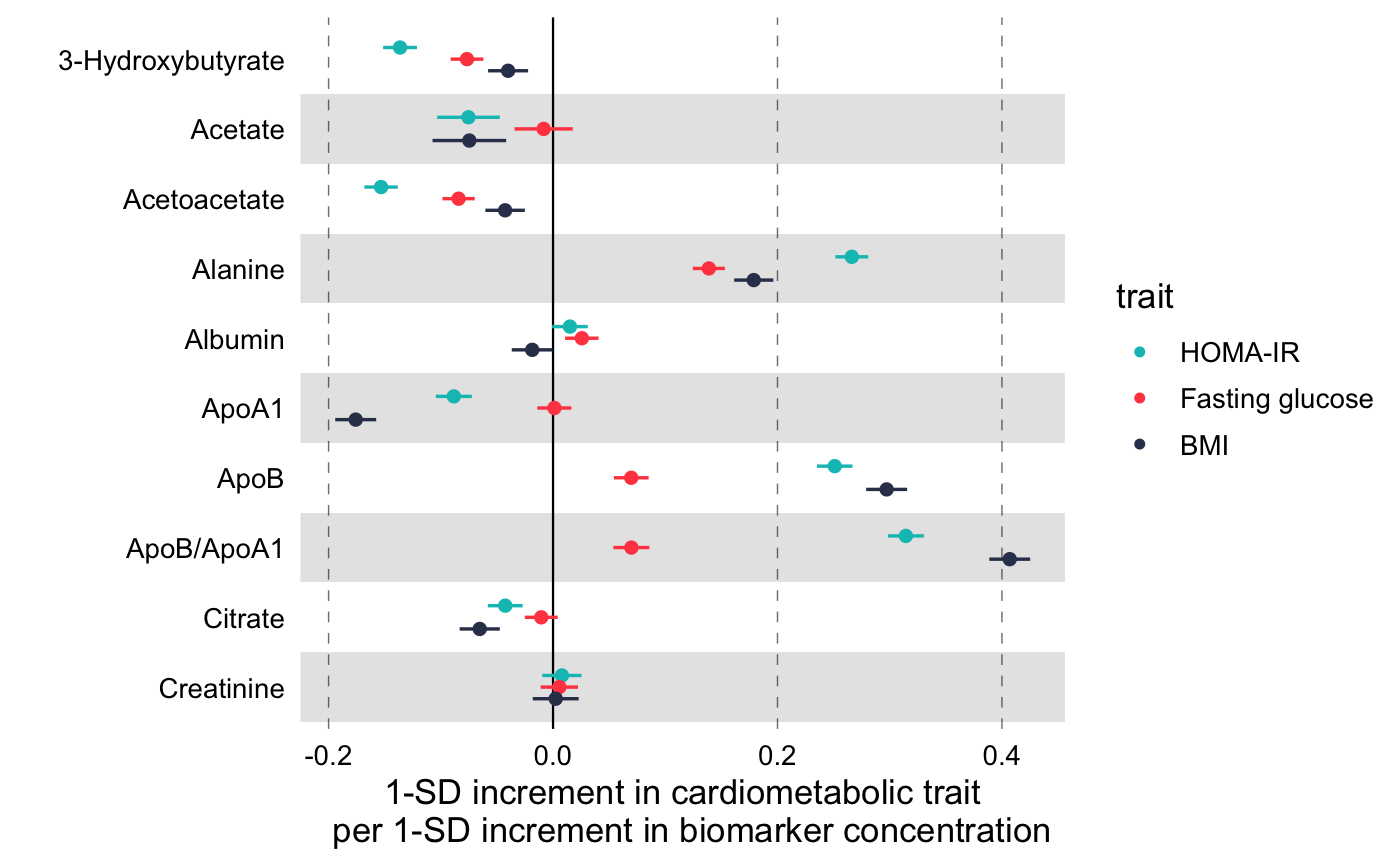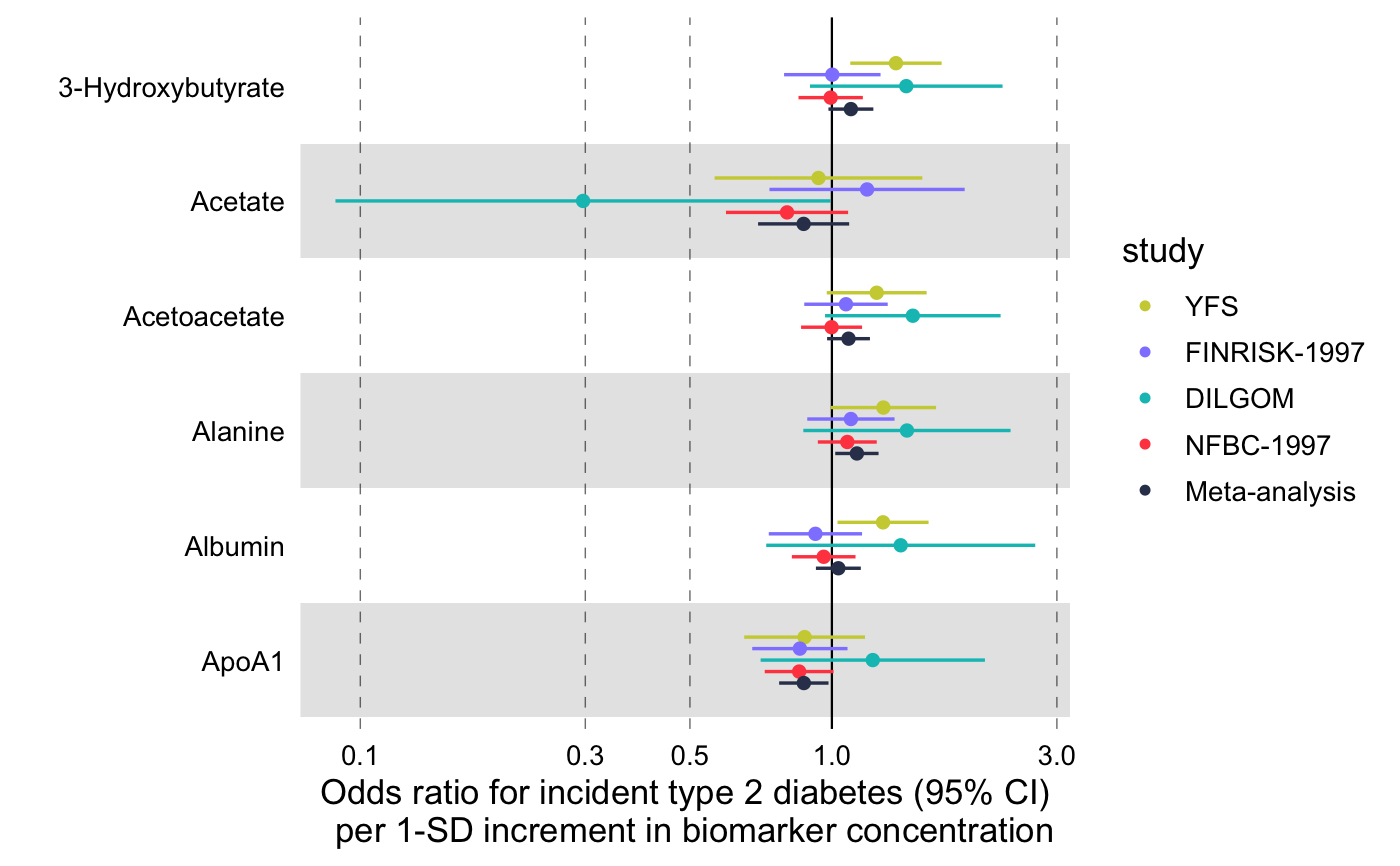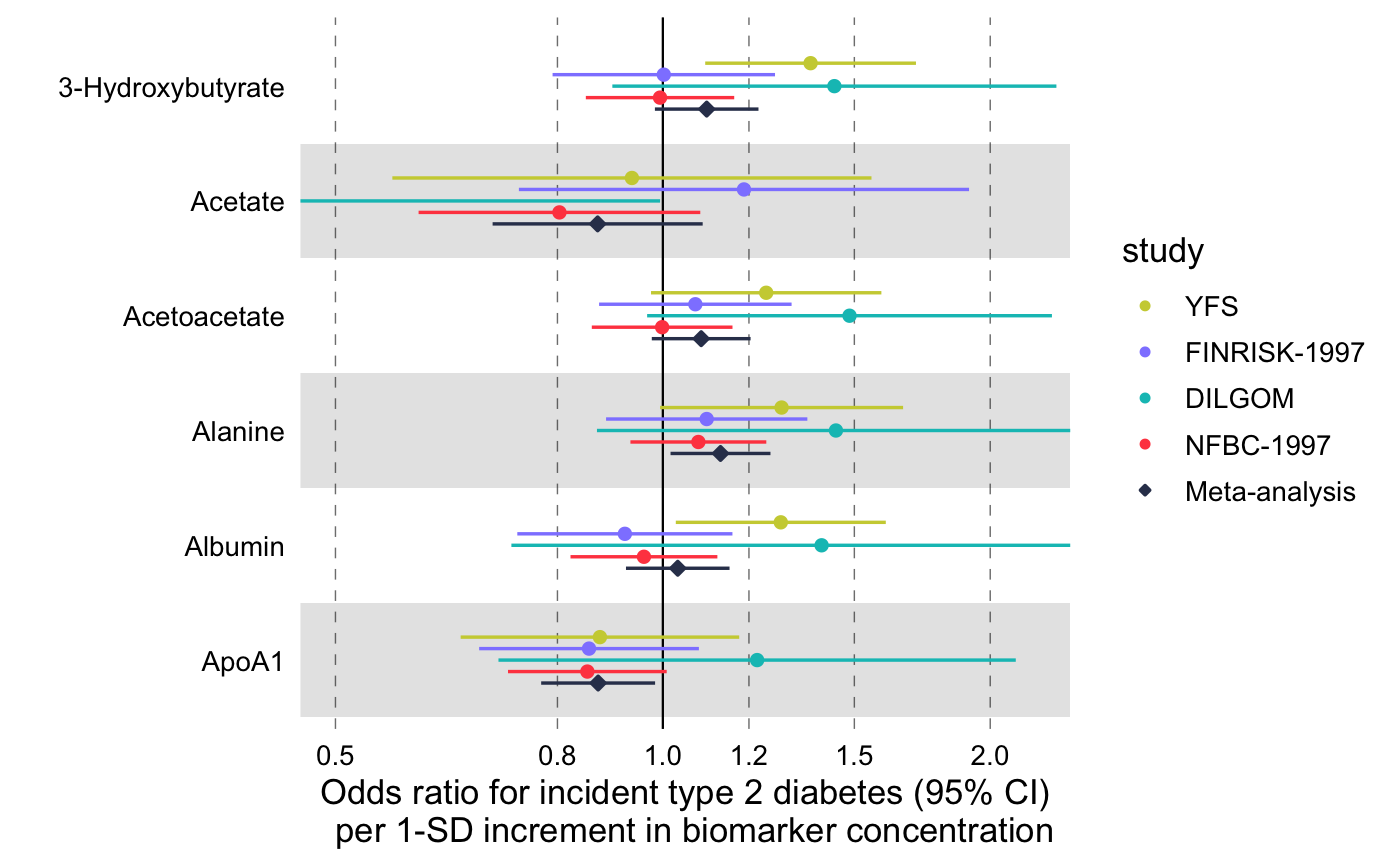Visualize multiple measures of effect with their confidence intervals in a vertical layout.

forestplot(
df,
name = name,
estimate = estimate,
se = se,
pvalue = NULL,
colour = NULL,
shape = NULL,
logodds = FALSE,
psignif = 0.05,
ci = 0.95,
...
)

## Arguments

df A data frame with the data to plot. It must contain at least three variables, a character column with the names to be displayed on the y-axis (see parameter name), a numeric column with the value (or the log of the value) to display (see parameter estimate) and a numeric value with the corresponding standard errors (see parameter se). It may contain additional columns, e.g. the corresponding p-values (see parameter pvalue) in which case, in conjuction with the threshold given in psignif, the non-significant results will be displayed as hollow points. Other variables may be used as aesthetics to define the colour and the shape of the points to be plotted. the variable in df that contains the y-axis names. This argument is automatically quoted and evaluated in the context of the df data frame. See Note. the variable in df that contains the values (or log of values) to be displayed. This argument is automatically quoted and evaluated in the context of the df data frame. See Note. the variable in the df data frame that contains the standard error values. This argument is automatically quoted and evaluated in the context of the df data frame. See Note. the variable in df that contains the p-values. Defaults to NULL. When explicitly defined, in conjuction with the p-value threshold provided in the psignif, the non-significant entries will be drawn as hollow points. This argument is automatically quoted and evaluated in the context of the df data frame. See Note. the variable in df by which to colour the different groups of points. This argument is automatically quoted and evaluated in the context of the df data frame. See Note. the variable in df by which to shape the different groups of points. This argument is automatically quoted and evaluated in the context of the df data frame. See Note. logical (defaults to FALSE) specifying whether the estimate parameter should be treated as log odds/hazards ratio (TRUE) or not (FALSE). When logodds = TRUE the estimates and corresponding confidence intervals will be exponentiated and a log scale will be used for the x-axis. numeric, defaults to 0.05. The p-value threshold for statistical significance. Entries with larger than psignif will be drawn with a hollow point. A number between 0 and 1 (defaults to 0.95) indicating the type of confidence interval to be drawn. ggplot2 graphical parameters such as title, ylab, xlab, xtickbreaks etc. to be passed along.

## Value

A ggplot object.

## Note

See vignette(programming, package = "dplyr") for an introduction to non-standard evaluation.

## Examples

library(magrittr)

# Linear associations
# Get subset of example data frame
df_linear <-
df_linear_associations %>%
dplyr::arrange(name) %>%
dplyr::filter(dplyr::row_number() <= 30)

# Forestplot
forestplot(
df = df_linear,
estimate = beta,
logodds = FALSE,
colour = trait,
xlab = "1-SD increment in cardiometabolic trait
per 1-SD increment in biomarker concentration"
)# Log odds ratios
df_logodds <-
df_logodds_associations %>%
dplyr::arrange(name) %>%
dplyr::filter(dplyr::row_number() <= 30) %>%
# Set the study variable to a factor to preserve order of appearance
# Set class to factor to set order of display.
dplyr::mutate(
study = factor(
study,
levels = c("Meta-analysis", "NFBC-1997", "DILGOM", "FINRISK-1997", "YFS")
)
)

# Forestplot
forestplot(
df = df_logodds,
estimate = beta,
logodds = TRUE,
colour = study,
xlab = "Odds ratio for incident type 2 diabetes (95% CI)
per 1-SD increment in biomarker concentration"
)# For the latter, if you want to restrain the x-axis and crop the large
# errorbar for Acetate you may add the following coord_cartesian layer
forestplot(
df = df_logodds,
estimate = beta,
logodds = TRUE,
colour = study,
shape = study,
xlab = "Odds ratio for incident type 2 diabetes (95% CI)
per 1-SD increment in biomarker concentration",
xlim = c(0.5, 2.2),
# You can explicitly define x-tick breaks
xtickbreaks = c(0.5, 0.8, 1.0, 1.2, 1.5, 2.0)
) +
# You may also want to add a manual shape to mark meta-analysis with a
# diamond shape
ggplot2::scale_shape_manual(
values = c(23L, 21L, 21L, 21L, 21L),
labels = c("Meta-analysis", "NFBC-1997", "DILGOM", "FINRISK-1997", "YFS")
)#> Scale for 'shape' is already present. Adding another scale for 'shape', which
#> will replace the existing scale.